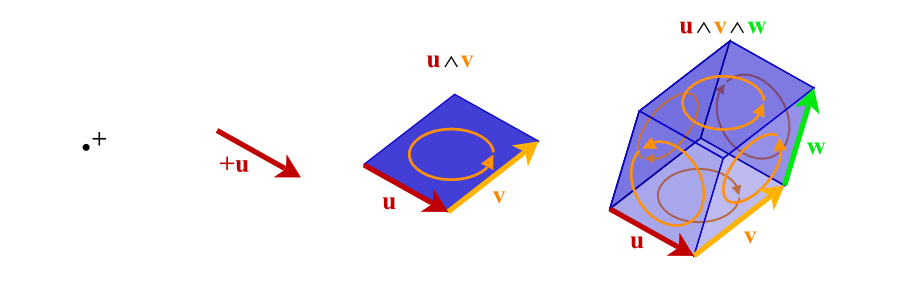# clifford: Geometric Algebra for Python¶

```In : from clifford.g3 import *  # import GA for 3D space

In : import math

In : a = e1 + 2*e2 + 3*e3  # vector

In : R = math.e**(math.pi/4*e12)  # rotor

In : R*a*~R    # rotate the vector
Out: (2.0^e1) - (1.0^e2) + (3.0^e3)
```

This module implements Geometric Algebras (a.k.a. Clifford algebras). Geometric Algebra (GA) is a universal algebra which subsumes complex algebra, quaternions, linear algebra and several other independent mathematical systems. Scalars, vectors, and higher-grade entities can be mixed freely and consistently in the form of mixed-grade multivectors.